# Arithmetic Operations in Pandas

## A guide on how to use arithmetic operations in Pandas.In this post, I'll show you how to apply arithmetic operations to the dataset and implement a function.

Let's dive in.

First of all, let's import the libraries.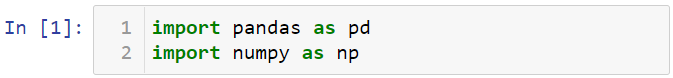Let me create two variables named s1 and s2.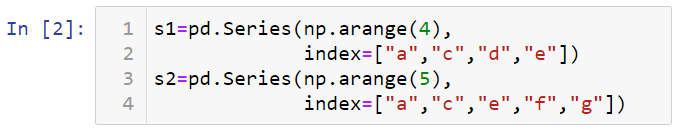Let's take a look at these variables.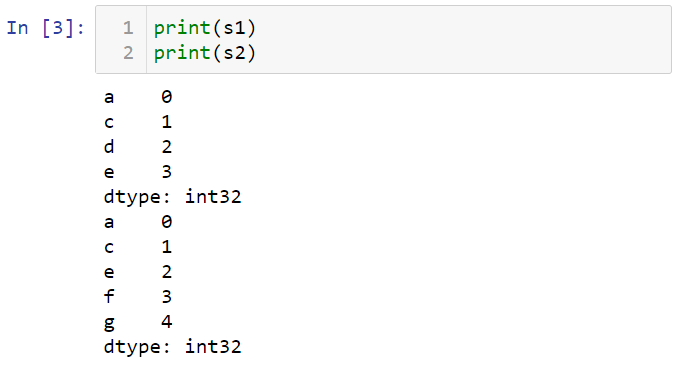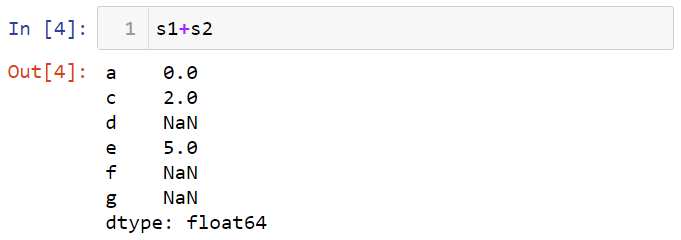You can do the same for the data frame. To show this, let's create two datasets named df1 and df2.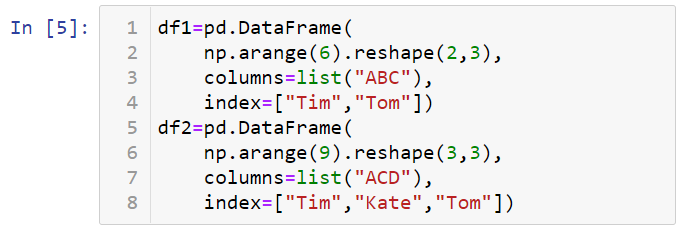Let's take a look at data frames.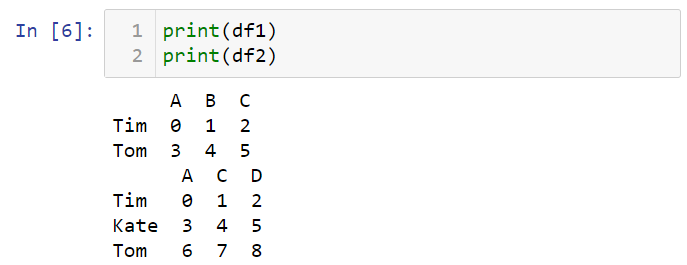You can add two data frames.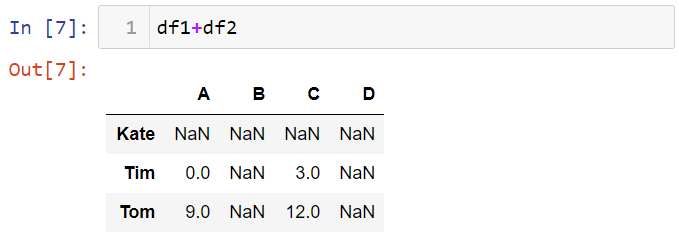When you can apply arithmetic operations with different indexes, a special value such as zero can be assigned to the missing values.

Let's assign zeros to values that do not match df2 of df1 when adding two data frames.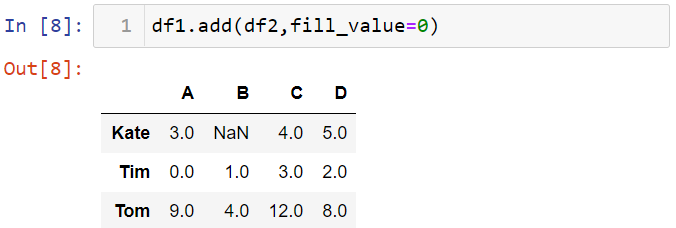### Multiplication & Division in Pandas

You can inverse the values in the dataset.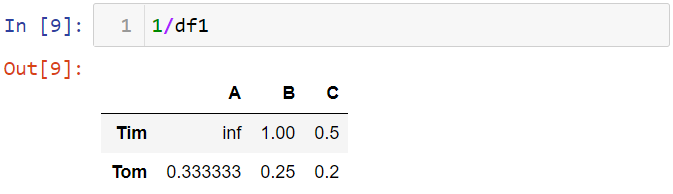Let me show multiplication.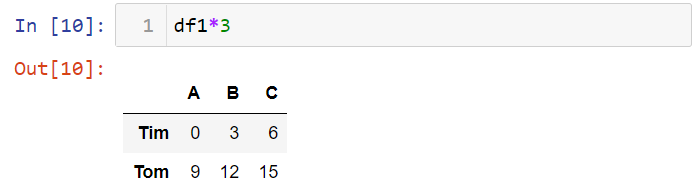You can also use the mul method for multiplication.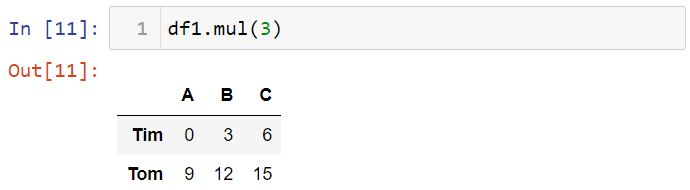I'm going to handle the df2 again. Let me print this data.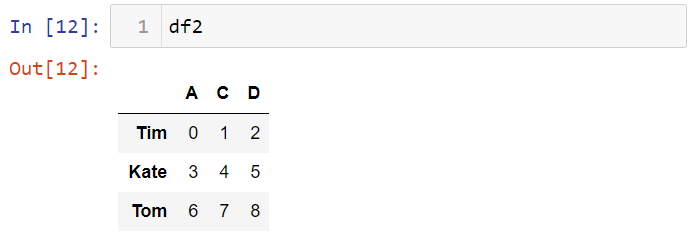Let's take a look at the first row of the df2.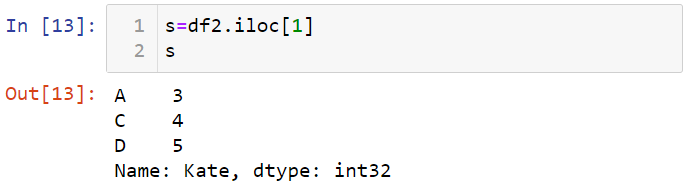As you can see, this row is in Serial structure. Let's subtract s from df2.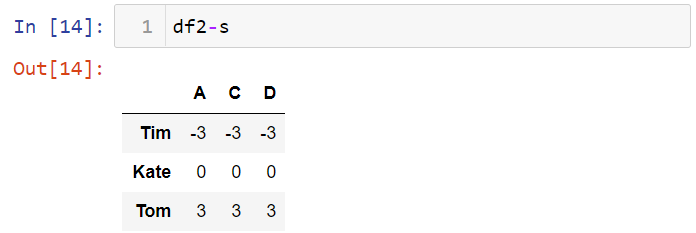To select a column in the dataset, you can use the [] symbol.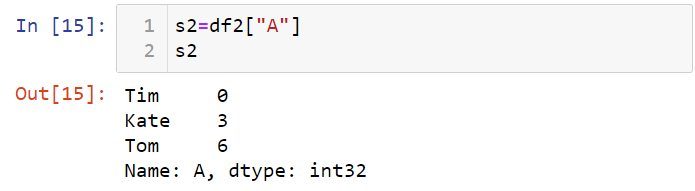You can perform arithmetic operations with a series and a data frame.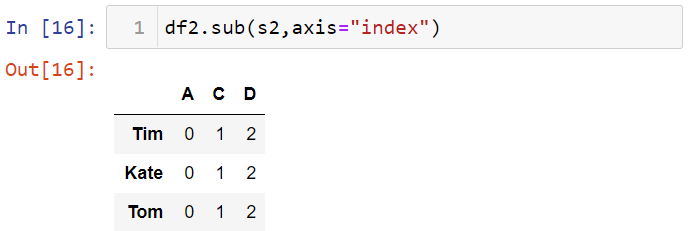### Applying a Function in Pandas

You can use NumPy functions with Pandas data structures To demonstrate this, let's first create a dataset named df.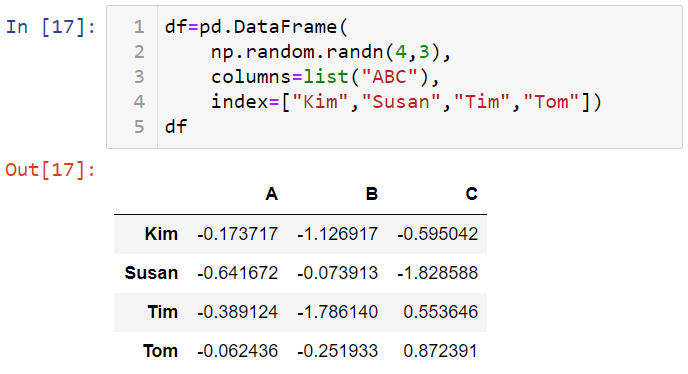Let's use the abs method in NumPy for df.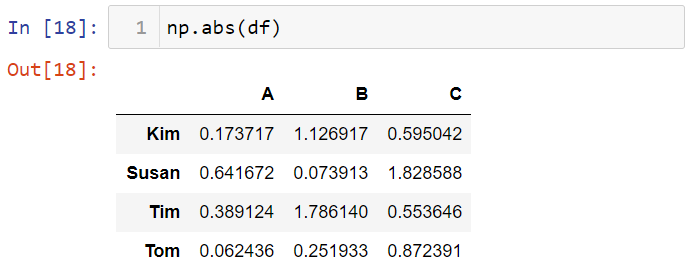You can also apply a function for each column or row. To do this, you can use the apply () method. First, let's create a function.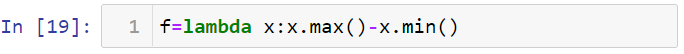Let's apply this function to the df using the apply method.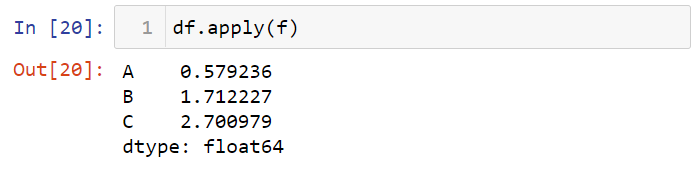You can execute this function on each row using axis = 1.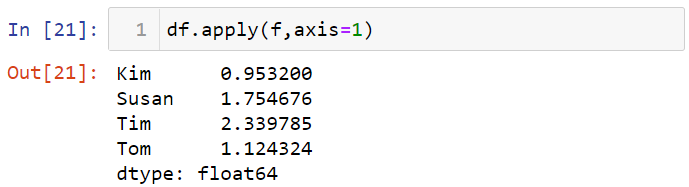You can use this function for the data frame. To show that, let's write a simple function.Now, let me apply this function to the df.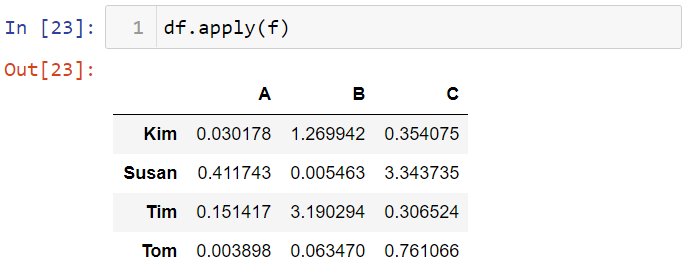That's it. I hope you enjoy this post. You can access the notebook here.